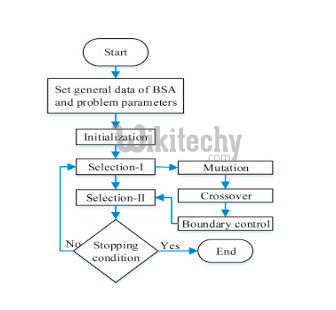# Server Hacking - Backtracking Algorithm

## Backtracking Algorithm

• Backtracking is algorithm for searching all solutions to computational problems, satisfaction problem, that creates candidates to the solutions.
• Backtracking is considered an important technique to solve constraint satisfaction issues and puzzles.

## General method:

• Useful technique for optimizing search under some constraints.
• Express the desired solution as an n-tuple (x1, . , xn) where each xi ∈ Si , Si being a finite set
• The solution is based on finding one or more vectors that maximize, minimize, or satisfy a criterion function P(x1, . . . , xn)
• Sorting of a[n] - Find an n-tuple where the element xi is the index of ith smallest element in a Criterion function is given by a[xi ] ≤ a[xi+1] for 1 ≤ i < n Set Si is a finite set of integers in the range [1,n]Backtracking Algorithm